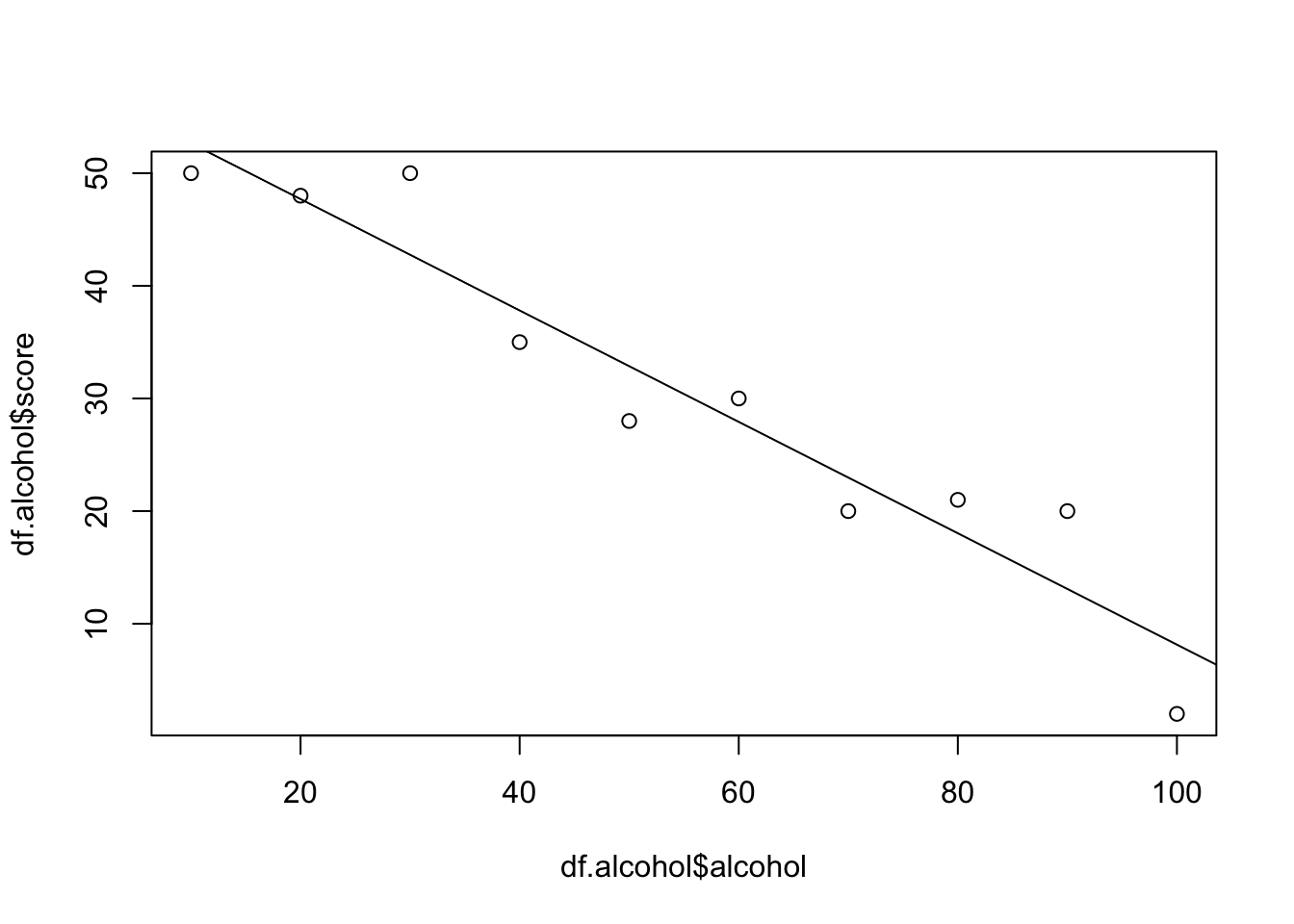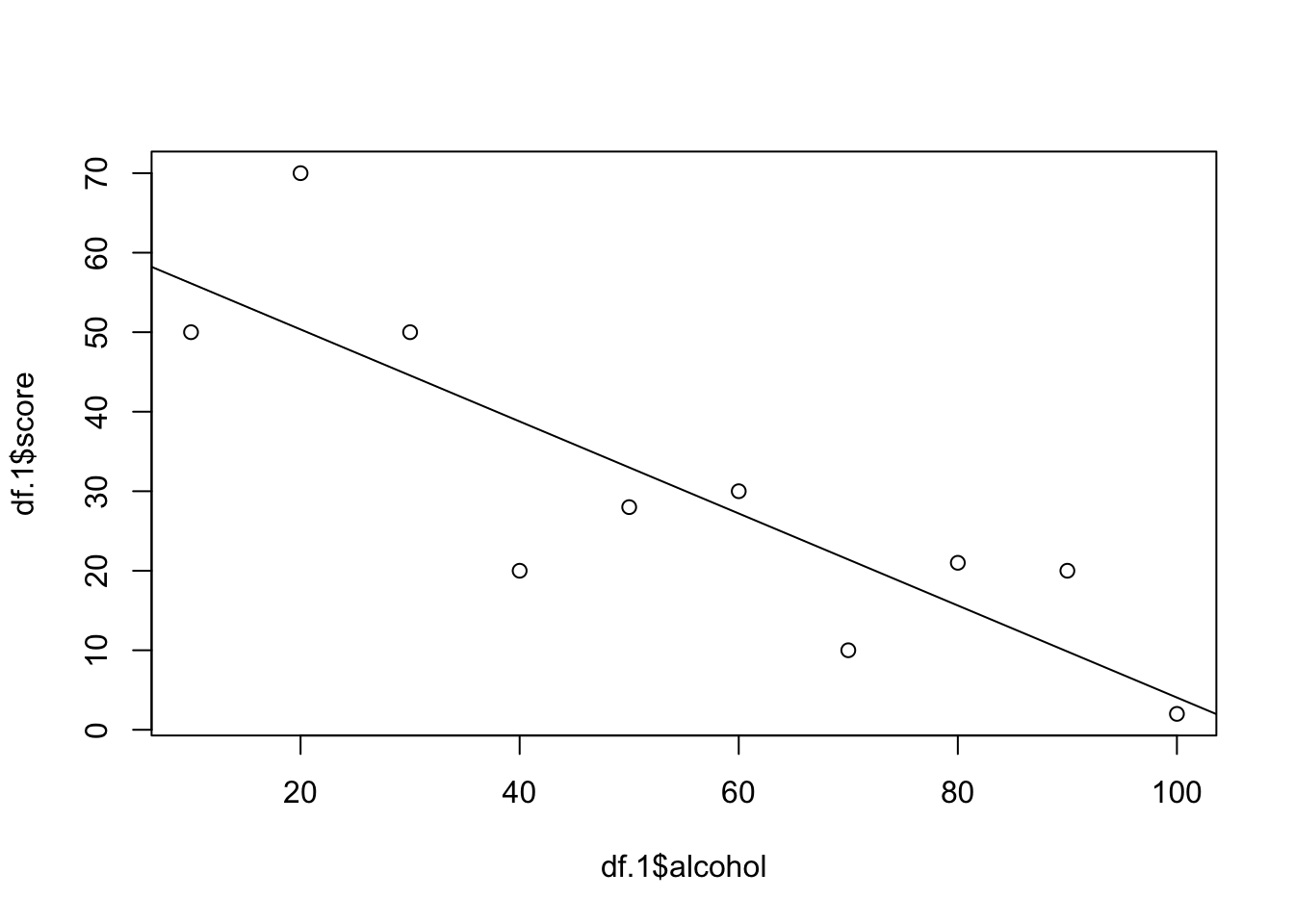Welcome to the second part of the workshop! Here we consider how to evaluate a model.

# Does this model look good on my data?

We have our hypothetical data from the last document and a linear model predicting the score outcome from the amount of alcohol in a drink.

``````rm(list=ls())
df.sweetner <- data.frame(sweetner = seq(10,100,10), score = c(50, 45, 55, 58, 55, 51, 59, 60, 50, 55))
df.alcohol <- data.frame(alcohol = seq(10,100,10), score = c(50, 48, 50, 35, 28, 30, 20, 21, 20, 2))
df.caffeine <- data.frame(caffeine = seq(10,100,10), score = c(42, 55, 60, 70, 68, 75, 78, 60, 50, 30))
m1 <- lm(score~ alcohol, data = df.alcohol)``````

## The fit

We know that our model misses some of the data point. That is to be expected.

``m1\$residuals``
``````##          1          2          3          4          5          6
## -2.6545455  0.2909091  7.2363636 -2.8181818 -4.8727273  2.0727273
##          7          8          9         10
## -2.9818182  2.9636364  6.9090909 -6.1454545``````

To examine how well our model fits the data we can look at the multiple R-squared (a single value!) in the summary output.

``summary(m1)``
``````##
## Call:
## lm(formula = score ~ alcohol, data = df.alcohol)
##
## Residuals:
##    Min     1Q Median     3Q    Max
## -6.146 -2.941 -1.182  2.741  7.236
##
## Coefficients:
##             Estimate Std. Error t value Pr(>|t|)
## (Intercept) 57.60000    3.40414  16.921 1.51e-07 ***
## alcohol     -0.49455    0.05486  -9.014 1.83e-05 ***
## ---
## Signif. codes:  0 '***' 0.001 '**' 0.01 '*' 0.05 '.' 0.1 ' ' 1
##
## Residual standard error: 4.983 on 8 degrees of freedom
## Multiple R-squared:  0.9104, Adjusted R-squared:  0.8992
## F-statistic: 81.26 on 1 and 8 DF,  p-value: 1.832e-05``````

Our Multiple R-squared is 0.9104. Is that good? In a bivariate regression the R-squared tells us the variation in the data accounted for in the model. In other words, the fit of our model to the data. this is not the true in multiple regression

What is R-squared? Well, R is the correlation coefficient between the predictions of the model and the actual data. At 1 the data and model predictions are the same. In our case, the R-squared value tells us that alcohol accounts for 90% of the variation in the score.

This is good. Both our plots of the model against the data (see below) and the R-squared value tell us our model captures the trend in the data, and this model uses the value of alcohol when creating predictions.

``````plot(df.alcohol\$alcohol, df.alcohol\$score)
abline(m1)``````## Uncertainty

Let us assume that our results are typical of people in general. This is quite an assumption and there are very legimitate reasons to not assume this (beyond the fact our study is completely hypothetical, of course). If our small sample is representative of the population then we can use our model to infer something about the population. In our case, consuming more alcohol makes us worse at arcade games.

But how certain are we about the contribution of alcohol to the score? We have a coefficient for alcohol that is -0.49455. This tells us that based on our model then one ml of alcohol decreases arcade games scores by 0.49455. But that value is a point estimate and considering the uncertaintly in this estimate is important. If we look at the summary of m1 we can see that each coefficient has a confidence interval.

``summary(m1)``
``````##
## Call:
## lm(formula = score ~ alcohol, data = df.alcohol)
##
## Residuals:
##    Min     1Q Median     3Q    Max
## -6.146 -2.941 -1.182  2.741  7.236
##
## Coefficients:
##             Estimate Std. Error t value Pr(>|t|)
## (Intercept) 57.60000    3.40414  16.921 1.51e-07 ***
## alcohol     -0.49455    0.05486  -9.014 1.83e-05 ***
## ---
## Signif. codes:  0 '***' 0.001 '**' 0.01 '*' 0.05 '.' 0.1 ' ' 1
##
## Residual standard error: 4.983 on 8 degrees of freedom
## Multiple R-squared:  0.9104, Adjusted R-squared:  0.8992
## F-statistic: 81.26 on 1 and 8 DF,  p-value: 1.832e-05``````

The standard Std. Error for the alcohol coefficient is quite small. If the data was more varied then we might expect a larger Std. Error for alcohol. Lets check.

``````df.1 <- data.frame(alcohol = seq(10,100,10), score = c(50, 70, 50, 20, 28, 30, 10, 21, 20, 2))
m2 <- lm(score~alcohol, data = df.1)
plot(df.1\$alcohol, df.1\$score)
abline(m2)````````summary(m2)``
``````##
## Call:
## lm(formula = score ~ alcohol, data = df.1)
##
## Residuals:
##      Min       1Q   Median       3Q      Max
## -18.7818  -5.8576   0.3697   5.4152  19.6424
##
## Coefficients:
##             Estimate Std. Error t value Pr(>|t|)
## (Intercept)  61.9333     8.0293   7.713 5.67e-05 ***
## alcohol      -0.5788     0.1294  -4.473  0.00208 **
## ---
## Signif. codes:  0 '***' 0.001 '**' 0.01 '*' 0.05 '.' 0.1 ' ' 1
##
## Residual standard error: 11.75 on 8 degrees of freedom
## Multiple R-squared:  0.7143, Adjusted R-squared:  0.6786
## F-statistic: 20.01 on 1 and 8 DF,  p-value: 0.002076``````

What do we see? As expected, in the new data there is more uncertainty about the value we should add to alcohol (the alcohol coefficient) to predict score.

We can also calculate the a confidence interval around our regression line. The confidence interval show us the range of values of score for each value of alcohol where the true regression line lies. Where many of the values are far from the line then the confidence interval will be greatest. We will use the 95% confidence interval and can calculate this with the predict function.

``````new.data <- data.frame(alcohol = seq(10,100,1)) # value we will calculate the confidence interval for
m2.ci <- predict(m2, newdata = new.data, interval = "confidence", level = 0.95)
m2.ci <- as.data.frame(m2.ci) # makes it easier to pick the coluns using syntax we already know
head(m2.ci) #first few fits and upper and lower condifence interval values``````

These upper and lower confidence values can be plotted on the same plot as the data.

For our poor fit data

``````plot(c(0,100), c(0,80), type='n', xlab = 'Alcohol (ml)', ylab = 'Score', main = 'Poorly fit alcohol data')
points(df.1\$alcohol, df.1\$score, col='black', pch=19)
abline(m2, col = 'red')
lines(new.data\$alcohol, m2.ci\$lwr, col="blue", lty=2)
lines(new.data\$alcohol, m2.ci\$upr, col="blue", lty=2)``````